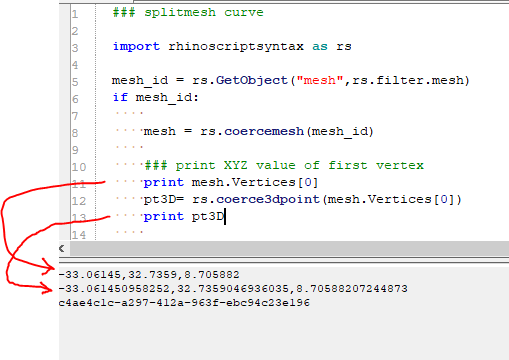# Mesh.Vertices accuracy seems wrong, why?

Hi guys, I am working on some mesh scripts and in evaluations of the vertices I wanted to coerce a point to check against, and then discovered that the vertex is vastly simplified compared to the coerced 3d point of that same vertex, why is that??? It seems very wrong that the mesh returns a lesser version of the vertex than a coerced version of the same point.

(The mesh is just a 100x100 mesh generated from a height field image, close to origin, nothing done to it, so it is as pure as can be)Hi @Holo,

Could be that the data type for the x, y, z components of a Point3d simply allows for a greater precision (more decimal places) than the data type of mesh vertex components?
Or it’s just the output rounding to 5 decimal places in the first case and printing all available decimal places in the second case?
Either way, I wouldn’t worry about this too much, since you probably won’t need that much precision.If you for instance need to compare points in your script, simply do so with a tolerance, which by the way is always a good idea in Rhino.Thanks, but that doesn’t make sense to me since the 3D point IS from the vertex, so it should not be able to add inn more detail… If it was just a bunch of 00000 filling up the space, then OK, but it isn’t. And that’s what bothers me. I want the raw data and expected the vertex to show it’s actual position. After all I am coercing that exact vertex, so what I coerce should be what the vertex IS and when I ask for that vertex I should get the same result as if I coerce it. (We work in Meters, so 5 digits are OK since that gives me 1/100 mm accuracy, I don’t need more than that, BUT if I run a " is coercedPt0 == vertises" then I don’t expect a False with out me having to run round(coercedPt0,5) for the statement to be True.

It is not a mistake.
Rhino records mesh vertices as a single point precision numbers:

So the second value that you see is probably conversion of the single point precision to double (called “float” in python).

As pirate suggested you should always use tolerance when comparing two numbers.

OK, but what I don’t understand is how coercing that point can add more data?
My understanding must be missing something here… Maybe how floating points work.

Does it make sense to you that the vertex returns x.xxxxx , y.yyyyy , z.zzzzz and that this once coerced into a 3d point is not x.xxxxx00000 , y.yyyyy00000 , z.zzzzz00000 ?

As mentioned multiple times on this forum don’t assume bit-for-bit parity when working with floating point numbers (single or duouble precision).

Further, Python does its own things to numbers when printing, so don’t rely on that either.

What may make sense to you as a normal human being does no longer hold in the realm of floating point computation and even just floating point representation in computer memory.

You can use the EpsilonEquals() utility functions provided by Rhino.RhinoMath:

https://developer.rhino3d.com/api/RhinoCommon/html/T_Rhino_RhinoMath.html

Ouch, you are right, that hurts my normal way of thinking and calculating numbers… don’t know if I want to tumble down that rabbit hole…But I still don’t understand why coercing the vertex doesn’t return the same number as just pringing the vertex value… why are they handled differently? Why is the vertex rounded when presented to me?

Here some more info on Python side of things - may be a bit different for IronPython, but probably holds for the most part:

https://docs.python.org/3/tutorial/floatingpoint.html

I recorded RH-58628 to keep track of this problem.

Right now, users can use the mesh.Vertices.GetPoint3dAt() function to look at the double precision vertex of the mesh. This is however a little less easy to find than the indexer `[]`, which returns the single precision.

2 Likes

From the Python floating point tutorial perhaps my favourite bit is this:

`print ( 0.1 + 0.1 + 0.1 == 0.3)`

Just paste that in EditPythonEditor and run it3 LikesOk, I am logging off and will stare at the sky for a while.
My world just crumbled.

1 Like

Welcome to the wondrous world of programming!

2 Likes

OK so I guess that with my new “knowledge” it refines to a wish:
I wish that the coerced 3D point of the single precision indexed point results in the same value as the printed value of that given point.
(Is that accurately stated? I feel I know nothing now…)

Printing single precision, as you can see, has less accuracy than double precision.
We can’t have the indexer return doubles now, because it would break existing plug-ins. And we are trying very hard to keep new versions of RhinoCommon compatible with older plug-ins.

We could deprecate the indexer, and print a warning that points to this or a similar discussion. It could also suggest the `GetPoint3dAt` method. Would that be enough?I don’t know anyting any more… Nathan just broke my mind…

``````A = (0.1+0.1+0.1)
B = 0.3
print A == B
``````

It’s False… Hahahahahaaa…

Give it a few days2 Likes

Maybe that is what happens to the binary value.
And then the same (binary) number is simply formatted as decimal using different precisions …
… I guess since one is a 32 bit value and the other a 64 bit value.

EDIT:

EDIT:

You may want to use the “r” (roundtrip) format specifier:
https://docs.microsoft.com/en-us/dotnet/standard/base-types/standard-numeric-format-strings

1 Like

After 20 years of math,geometry programming I ended up to think geometry like what happens in the real world, a point a line and a surface do not exist. They are balls, cylinders and skins with a epsilon radius/thickness. This helps to make robust coding (at least I hope)
Gd